# Rosetta¶

Contents:

## All Modules and Classes¶

### cmd¶

Unix-like command line utilities. Filters (read from stdin/write to stdout) for files

Installation should put these in your path. To see help, do

module_name.py -h


### parallel¶

• Wrappers for Python multiprocessing that add ease of use
• Memory-friendly multiprocessing

#### parallel_easy¶

Functions to assist in parallel processing with Python 2.7.

• Memory-friendly iterator functionality (wrapping Pool.imap).
• Exit with Ctrl-C.
• Easy use of n_jobs. (e.g. when n_jobs == 1, processing is serial)
• Similar to joblib.Parallel but with the addition of imap functionality and a more effective way of handling Ctrl-C exit (we add a timeout).
rosetta.parallel.parallel_easy.imap_easy(func, iterable, n_jobs, chunksize, ordered=True)[source]

Returns a parallel iterator of func over iterable.

Worker processes return one “chunk” of data at a time, and the iterator allows you to deal with each chunk as they come back, so memory can be handled efficiently.

Parameters: func : Function of one variable You can use functools.partial to build this. A lambda function will not work iterable : List, iterator, etc... func is applied to this n_jobs : Integer The number of jobs to use for the computation. If -1 all CPUs are used. If 1 is given, no parallel computing code is used at all, which is useful for debugging. For n_jobs below -1, (n_cpus + 1 + n_jobs) are used. Thus for n_jobs = -2, all CPUs but one are used. chunksize : Integer Jobs/results will be sent between master/slave processes in chunks of size chunksize. If chunksize is too small, communication overhead slows things down. If chunksize is too large, one process ends up doing too much work (and large results will up in memory). ordered : Boolean If True, results are dished out in the order corresponding to iterable. If False, results are dished out in whatever order workers return them.

Examples

>>> from functools import partial
>>> from rosetta.parallel.parallel_easy import imap_easy
>>> def abfunc(x, a, b=1):
...     return x * a * b
>>> some_numbers = range(3)
>>> func = partial(abfunc, 2, b=3)
>>> results_iterator = imap_easy(func, some_numbers, 2, 5)
>>> for result in results_iterator:
...     print result
0
6
12

rosetta.parallel.parallel_easy.map_easy(func, iterable, n_jobs)[source]

Returns a parallel map of func over iterable. Returns all results at once, so if results are big memory issues may arise

Parameters: func : Function of one variable You can use functools.partial to build this. A lambda function will not work iterable : List, iterator, etc... func is applied to this n_jobs : Integer The number of jobs to use for the computation. If -1 all CPUs are used. If 1 is given, no parallel computing code is used at all, which is useful for debugging. For n_jobs below -1, (n_cpus + 1 + n_jobs) are used. Thus for n_jobs = -2, all CPUs but one are used.

Examples

>>> from functools import partial
>>> from rosetta.parallel.parallel_easy import map_easy
>>> def abfunc(x, a, b=1):
...     return x * a * b
>>> some_numbers = range(5)
>>> func = partial(abfunc, 2, b=3)
>>> map_easy(func, some_numbers)
[0, 6, 12, 18, 24]

rosetta.parallel.parallel_easy.map_easy_padded_blocks(func, iterable, n_jobs, pad, blocksize=None)[source]

Returns a parallel map of func over iterable, computed by splitting iterable into padded blocks, then piecing the result together.

Parameters: func : Function of one variable You can use functools.partial to build this. A lambda function will not work iterable : List, iterator, etc... func is applied to this n_jobs : Integer The number of jobs to use for the computation. If -1 all CPUs are used. If 1 is given, no parallel computing code is used at all, which is useful for debugging. For n_jobs below -1, (n_cpus + 1 + n_jobs) are used. Thus for n_jobs = -2, all CPUs but one are used. pad : Nonnegative Integer Each block is processed with pad extra on each side. blocksize : Nonnegative Integer If None, use 100 * pad result : List Equivalent to list(func(iterable))

Examples

>>> numbers = [0, 0, 2, -1, 4, 2, 6, 7, 6, 9]
>>> n_jobs = -1
>>> def rightmax(mylist):
...     return [max(mylist[i: i+2]) for i in range(len(mylist))]
>>> benchmark = rightmax(numbers)
>>> result == benchmark
True

rosetta.parallel.parallel_easy.parallel_apply(func, iterable, n_jobs, sep='\n', out_stream=<open file '<stdout>', mode 'w'>)[source]

Writes the result of applying func to iterable using n_jobs to out_stream

#### pandas_easy¶

Functions for helping make pandas parallel.

rosetta.parallel.pandas_easy.groupby_to_scalar_to_series(df_or_series, func, n_jobs, **groupby_kwargs)[source]

Returns a parallelized, simplified, and restricted version of: df_or_series.groupby(**groupby_kwargs).apply(func)

Works ONLY for the simple case that .apply(func) would yield a Series of length equal to the number of groups, in other words, func applied to each group is a scalar.

Parameters: df_or_series : DataFrame or Series This is what is grouped func : Function Applied to each group using func(df_or_series) Should return one single value (e.g. string or number) Must be picklable: A lambda function will not work! groupby_kwargs : Keyword args Passed directly to DataFrame.groupby to determine groups. The most common one is “by”, e.g. by=’a’ by=my_grouper_function by=my_grouping_list_of_labels result : Series Index is the group names Values are func(group) iterated over every group

Examples

>>> from rosetta.parallel.pandas_easy import groupby_to_series
>>> df = pd.DataFrame({'a': [6, 2, 2], 'b': [4, 5, 6]})
>>> df
a  b
0  6  4
1  2  5
2  2  6
>>> groupby_to_series(df, max, n_jobs, by='a')
2    b
6    b

>>> s = pd.Series([1, 2, 3, 4])
>>> s
0    1
1    2
2    3
3    4
>>> labels = ['a', 'a', 'b', 'b']
>>> groupby_to_series(s, max, 1, by=labels)
a    2
b    4

rosetta.parallel.pandas_easy.groupby_to_series_to_frame(frame, func, n_jobs, use_apply=True, **groupby_kwargs)[source]

A parallel function somewhat similar DataFrame.groupby.apply(func).

For each group in df_or_series.groupby(**groupby_kwargs), compute func(group) or group.apply(func) and, assuming each result is a series, flatten each series then paste them together.

Parameters: frame : DataFrame func : Function Applied to each group using func(df_or_series) Must be picklable: A lambda function will not work! use_apply : Boolean If True, use group.apply(func) If False, use func(group) groupby_kwargs : Keyword args Passed directly to DataFrame.groupby to determine groups. The most common one is “by”, e.g. by=’a’ by=my_grouper_function by=my_grouping_list_of_labels result : DataFrame Index is the group names Values are func(group) iterated over every group, then pasted together

Examples

>>> from rosetta.parallel.pandas_easy import groupby_to_series_to_frame
>>> df = pd.DataFrame({'a': [6, 2, 2], 'b': [4, 5, 6]})
>>> labels = ['g1', 'g1', 'g2']
# Result and benchmark will be equal...despite the fact that you can't
# do df.groupby(labels).apply(np.mean)
>>> benchmark = df.groupby(labels).mean()
>>> result = groupby_to_series_to_frame(
...    df, np.mean, 1, use_apply=True, by=labels)
>>> print result
a    b
g1  4  4.5
g2  2  6.0


### text¶

Text-processing specific

• Stream text from disk to formats used in common ML processes
• Write processed text to sparse formats
• Helpers for ML tools (e.g. Vowpal Wabbit, Gensim, etc...)
• Other general utilities

#### filefilter¶

Contains a collection of function that clean, decode and move files around.

class rosetta.text.filefilter.PathFinder(text_base_path=None, file_type='*', name_strip='\..*', limit=None)[source]

Find and access paths in a directory tree.

rosetta.text.filefilter.get_paths(base_path, file_type='*', relative=False, get_iter=False, limit=None)[source]

Crawls subdirectories and returns an iterator over paths to files that match the file_type.

Parameters: base_path : String Path to the directory that will be crawled file_type : String String to filter files with. E.g. ‘*.txt’. Note that the filenames will be converted to lowercase before this comparison. relative : Boolean If True, get paths relative to base_path If False, get absolute paths get_iter : Boolean If True, return an iterator over paths rather than a list.
rosetta.text.filefilter.path_to_name(path, strip_ext=True)[source]

Takes one path and returns the filename, excluding the extension.

rosetta.text.filefilter.path_to_newname(path, name_level=1)[source]

Takes one path and returns a new name, combining the directory structure with the filename.

Parameters: path : String name_level : Integer Form the name using items this far back in the path. E.g. if path = mydata/1234/3.txt and name_level == 2, then name = 1234_3 name : String

#### streamers¶

Classes for streaming tokens/info from files/sparse files etc...

class rosetta.text.streamers.BaseStreamer[source]

Base class...don’t use this directly.

Methods

info_stream(**kwargs)[source]

Abstract method. All derived classes will implement to return an interator over the text documents with processing as appropriate.

single_stream(item, cache_list=None, **kwargs)[source]

Stream a single item from source.

Parameters: item : String The single item to pull from info and stream. cache_list : List of strings Cache these items on every iteration kwargs : Keyword args Passed on to self.info_stream
to_scipysparse(cache_list=None, **kwargs)[source]

Returns a scipy sparse matrix representing the collection of documents as a bag of words, one (sparse) row per document. Translation from column to token are stored into the cache

Parameters: cache_list : List of strings. Cache these items as they appear E.g. self.token_stream(‘doc_id’, ‘tokens’) caches info[‘doc_id’] and info[‘tokens’] (assuming both are available). kwargs : Keyword args Passed on to self.info_stream
to_vw(out_stream=<open file '<stdout>', mode 'w'>, n_jobs=1, raise_on_bad_id=True, cache_list=None)[source]

Write our filestream to a VW (Vowpal Wabbit) formatted file.

Parameters: out_stream : stream, buffer or open file object n_jobs : Integer number of CPU jobs cache_list : List of strings. Cache these items as they appear E.g. self.token_stream(‘doc_id’, ‘tokens’) caches info[‘doc_id’] and info[‘tokens’] (assuming both are available). Notes: : —– : self.info_stream must have a ‘doc_id’ field, as this is used to index : the lines in the vw file. :
token_stream(cache_list=None, **kwargs)[source]

Returns an iterator over tokens with possible caching of other info.

Parameters: cache_list : List of strings. Cache these items as they appear E.g. self.token_stream(‘doc_id’, ‘tokens’) caches info[‘doc_id’] and info[‘tokens’] (assuming both are available). kwargs : Keyword args Passed on to self.info_stream
class rosetta.text.streamers.DBStreamer(db_setup, tokenizer=None, tokenizer_func=None)[source]

Database streamer base class

Methods

connect()[source]

Open connection to database. sets the classes cursor object.

disconnect()[source]

Close connection to database

info_stream()[source]

Yields a dict from self.executing the query as well as “tokens”.

iterate_over_query()[source]

Return an iterator over query result. We suggest that the entire query result not be returned and that iteration is controlled on server side, but this method does not guarantee that. This method must return a dictionary, which at least has the key ‘text’ in it, containing the next to be tokenized.

class rosetta.text.streamers.MongoStreamer(db_setup, tokenizer=None, tokenizer_func=None)[source]

Subclass of DBStreamer to connect to a Mongo database and iterate over query results. db_setup is expected to be a dictionary containing host, database, collection, query, and text_key. Additionally an optional limit parameter is allowed. The query itself must return a column named text_key which is passed on as ‘text’ to the iterator. In addition, because it is difficult to rename mongo fields (similar to the SQL ‘AS’ syntax), we allow a translation dictionary to be passed in, which translates keys in the mongo dictionary result names k to be passed into the result as v for key value pairs {k : v}. Currently we don’t deal with nested documents.

Example:

db_setup = {} db_setup[‘host’] = ‘localhost’ db_setup[‘database’] = ‘places’ db_setup[‘collection’] = ‘opentable’ db_setup[‘query’] = {} db_setup[‘limit’] = 5 db_setup[‘text_key’] = ‘desc’ db_setup[‘translations’] = {‘_id’ : ‘doc_id’}

# In this example, we assume that the collection has a field named # desc, holding the text to be analyzed, and a field named _id which # will be translated to doc_id and stored in the cache.

my_tokenizer = TokenizerBasic() stream = MongoStreamer(db_setup=db_setup, tokenizer=my_tokenizer)

for text in stream.info_stream(cache_list=[‘doc_id’]):
print text[‘doc_id’], text[‘tokens’]

Methods

class rosetta.text.streamers.MySQLStreamer(*args, **kwargs)[source]

Subclass of DBStreamer to connect to a MySQL database and iterate over query results. db_setup is expected to be a dictionary containing host, user, password, database, and query. The query itself must return a column named text.

Example:

db_setup = {} db_setup[‘host’] = ‘hostname’ db_setup[‘user’] = ‘username’ db_setup[‘password’] = ‘password’ db_setup[‘database’] = ‘database’ db_setup[‘query’] = ‘select

id as doc_id, body as text

from tablename where length(body) > 100’

my_tokenizer = TokenizerBasic() stream = MySQLStreamer(db_setup=db_setup, tokenizer=my_tokenizer)

for text in stream.info_stream(cache_list=[‘doc_id’]):
print text[‘doc_id’], text[‘tokens’]

Methods

class rosetta.text.streamers.TextFileStreamer(text_base_path=None, path_list=None, file_type='*', name_strip='\..*', tokenizer=None, tokenizer_func=None, limit=None, shuffle=True)[source]

For streaming from text files.

Methods

info_stream(paths=None, doc_id=None, limit=None)[source]

Returns an iterator over paths yielding dictionaries with information about the file contained within.

Parameters: paths : list of strings doc_id : list of strings or ints limit : Integer Use limit in place of self.limit.
to_vw(outfile, n_jobs=1, chunksize=1000, raise_on_bad_id=True)[source]

Write our filestream to a VW (Vowpal Wabbit) formatted file.

Parameters: outfile : filepath or buffer n_jobs : Integer Use n_jobs different jobs to do the processing. Set = 4 for 4 jobs. Set = -1 to use all available, -2 for all except 1,... chunksize : Integer Workers process this many jobs at once before pickling and sending results to master. If this is too low, communication overhead will dominate. If this is too high, jobs will not be distributed evenly. raise_on_bad_id : Boolean If True, raise DocIDError when the doc_id (formed by self) is not a valid VW “Tag”. I.e. contains :, |, ‘, or whitespace. If False, print warning.
class rosetta.text.streamers.TextIterStreamer(text_iter, tokenizer=None, tokenizer_func=None)[source]

For streaming text.

Methods

info_stream()[source]

Yields a dict from self.streamer as well as “tokens”.

record_stream()[source]

Yields a dict from self.streamer

class rosetta.text.streamers.VWStreamer(sfile=None, cache_sfile=False, limit=None, shuffle=False)[source]

For streaming from a single VW file. Since the VW file format does not preserve token order, all tokens are unordered.

Methods

info_stream(doc_id=None)[source]

Returns an iterator over info dicts.

Parameters: doc_id : Iterable over Strings Return info dicts iff doc_id in doc_id
record_stream(doc_id=None)[source]

Returns an iterator over record dicts.

Parameters: doc_id : Iterable over Strings Return record dicts iff doc_id in doc_id

#### text_processors¶

##### Tokenizer¶

Classes with a .text_to_token_list method (and a bit more). Used by other modules as a means to convert stings to lists of strings.

If you have a function that converts strings to lists of strings, you can make a tokenizer from it by using MakeTokenizer(my_tokenizing_func).

##### SparseFormatter¶

Classes for converting text to sparse representations (e.g. VW or SVMLight).

##### SFileFilter¶

Classes for filtering words/rows from a sparse formatted file.

class rosetta.text.text_processors.BaseTokenizer[source]

Base class, don’t use directly.

Methods

text_to_counter(text)[source]

Return a counter associated to tokens in text. Filter/transform words according to the scheme this Tokenizer uses.

Parameters: text : String tokens : Counter keys = the tokens values = counts of the tokens in text
class rosetta.text.text_processors.MakeTokenizer(tokenizer_func)[source]

Makes a subclass of BaseTokenizer out of a function.

Methods

class rosetta.text.text_processors.SFileFilter(formatter, bit_precision=18, sfile=None, verbose=True)[source]

Filters results stored in sfiles (sparsely formattted bag-of-words files).

Methods

compactify()[source]

Removes “gaps” in the id values in self.token2id. Every single id value will (probably) be altered.

filter_extremes(doc_freq_min=0, doc_freq_max=inf, doc_fraction_min=0, doc_fraction_max=1, token_score_min=0, token_score_max=inf, token_score_quantile_min=0, token_score_quantile_max=1)[source]

Remove extreme tokens from self (calling self.filter_tokens).

Parameters: doc_freq_min : Integer Remove tokens that in less than this number of documents doc_freq_max : Integer doc_fraction_min : Float in [0, 1] Remove tokens that are in less than this fraction of documents doc_fraction_max : Float in [0, 1] token_score_quantile_min : Float in [0, 1] Minimum quantile that the token score (usually total token count) can be in. token_score_quantile_max : Float in [0, 1] Maximum quantile that the token score can be in self :
filter_sfile(infile, outfile, doc_id_list=None, enforce_all_doc_id=True, min_tf_idf=0, filters=None)[source]

Alter an sfile by converting tokens to id values, and removing tokens not in self.token2id. Optionally filters on doc_id, tf_idf and user-defined filters.

Parameters: infile : file path or buffer outfile : file path or buffer doc_id_list : Iterable over strings Keep only rows with doc_id in this list enforce_all_doc_id : Boolean If True (and doc_id is not None), raise exception unless all doc_id in doc_id_list are seen. min_tf_idf : int or float Keep only tokens whose term frequency-inverse document frequency is greater than this threshold. Given a token t and a document d in a corpus of documents D, tf_idf is given by the following formula: tf_idf(t, d, D) = tf(t, d) x idf(t, D), where tf(t, d) is the number of times the term t shows up in the document d, idf(t, D) = log (N / M), where N is the total number of documents in D and M is the number of documents in D which contain the token t. The logarithm is base e. filters : iterable over functions Each function must take a record_dict as a parameter and return a boolean. The record_dict may (and usually should) be altered in place. If the return value is False, the record_dict (corresponding to a document) is filtered out of the sfile. Both the doc_id_list and min_tf_idf parameters are implemented in this style internally. If the doc_id_list or min_tf_idf flags are set, those filters will run before the those found in filters. See rosetta/text/streaming_filters.py in the rosetta repository for the implementation details of the record_dict and built-in filters as well as explanations of how to define more filters.
filter_tokens(tokens)[source]

Remove tokens from appropriate attributes.

Parameters: tokens : String or iterable over strings E.g. a single token or list of tokens self :
load_sfile(sfile)[source]

Parameters: sfile : String or open file The sparse formatted file we will load. self :
save(savepath, protocol=-1, set_id2token=True)[source]

Pickle self to outfile.

Parameters: savefile : filepath or buffer protocol : 0, 1, 2, -1 0 < 1 < 2 in terms of performance. -1 means use highest available. set_id2token : Boolean If True, set self.id2token before saving. Used to associate tokens with the output of a VW file.
set_bit_precision_required()[source]

Sets self.bit_precision_required to the minimum bit precision b such that all token id values are less than 2^b.

The idea is that only compactification can change this, so we only (automatically) call this after compactification.

set_id2token(seed=None)[source]

Sets self.id2token, resolving collisions as needed (which alters self.token2id)

to_frame()[source]

Return a dataframe representation of self.

class rosetta.text.text_processors.SVMLightFormatter[source]

For formatting in/out of SVM-Light format (info not currently supported) http://svmlight.joachims.org/

<line> .=. <target> <feature>:<value> <feature>:<value> ... <target> .=. +1 | -1 | 0 | <float> <feature> .=. <integer> | “qid” <value> .=. <float> <info> .=. <string>

Methods

get_sstr(feature_values=None, target=1, importance=None, doc_id=None)[source]

Return a string reprsenting one record in SVM-Light sparse format <line> .=. <target> <feature>:<value> <feature>:<value>

Parameters: feature_values : Dict-like {hash1: value1,...} target : Real number The value we are trying to predict. formatted : String Formatted in SVM-Light
class rosetta.text.text_processors.SparseFormatter[source]

Base class for sparse formatting, e.g. VW or svmlight. Not meant to be directly used.

Methods

sfile_to_token_iter(filepath_or_buffer, limit=None)[source]

Return an iterator over filepath_or_buffer that returns, line-by-line, a token_list.

Parameters: filepath_or_buffer : string or file handle / StringIO. File should be formatted according to self.format. token_iter : Iterator E.g. token_iter.next() gets the next line as a list of tokens.
sstr_to_dict(sstr)[source]

Returns a dict representation of sparse record string.

Parameters: sstr : String String representation of one record. record_dict : Dict possible keys = ‘target’, ‘importance’, ‘doc_id’, ‘feature_values’

Notes

rstrips newline characters from sstr before parsing.

sstr_to_info(sstr)[source]

Returns the full info dictionary corresponding to a sparse record string. This holds “everything.”

Parameters: sstr : String String representation of one record. info : Dict possible keys = ‘tokens’, ‘target’, ‘importance’, ‘doc_id’, ‘feature_values’, etc...
sstr_to_token_list(sstr)[source]

Convertes a sparse record string to a list of tokens (with repeats) corresponding to sstr.

E.g. if sstr represented the dict {‘hi’: 2, ‘bye’: 1}, then token_list = [‘hi’, ‘hi’, ‘bye’] (up to permutation).

Parameters: sstr : String Formatted according to self.format_name Note that the values in sstr must be integers. token_list : List of Strings
class rosetta.text.text_processors.TokenizerBasic[source]

A simple tokenizer. Extracts word counts from text.

Keeps only non-stopwords, converts to lowercase, keeps words of length >=2.

Methods

text_to_token_list(text)[source]

Return a list of tokens. Filter/transform words according to the scheme this Tokenizer uses.

Parameters: text : String tokens : List Tokenized text, e.g. [‘hello’, ‘my’, ‘name’, ‘is’, ‘ian’]
class rosetta.text.text_processors.TokenizerPOSFilter(pos_types=[], sent_tokenizer=<function sent_tokenize>, word_tokenizer=<rosetta.text.text_processors.TokenizerBasic object>, word_tokenizer_func=None, pos_tagger=<function pos_tag>)[source]

Tokenizes, does POS tagging, then keeps words that match particular POS.

Methods

text_to_token_list(text)[source]

Tokenize a list of text that (possibly) includes multiple sentences.

class rosetta.text.text_processors.VWFormatter[source]

Converts in and out of VW format (namespaces currently not supported). Many valid VW inputs are possible, we ONLY support

[target] [Importance [Tag]]| feature1[:value1] feature2[:value2] ...

Every single whitespace, pipe, colon, and newline is significant.

Methods

get_sstr(feature_values=None, target=None, importance=None, doc_id=None)[source]

Return a string reprsenting one record in sparse VW format:

Parameters: feature_values : Dict-like {feature1: value1,...} target : Real number The value we are trying to predict. importance : Real number The importance weight to associate to this example. doc_id : Number or string A name for this example. formatted : String Formatted in VW format
rosetta.text.text_processors.collision_probability(vocab_size, bit_precision)[source]

Approximate probability of at least one collision (assuming perfect hashing). See the Wikipedia article on “The birthday problem” for details.

Parameters: vocab_size : Integer Number of unique words in vocabulary bit_precision : Integer Number of bits in space we are hashing to

#### nlp¶

rosetta.text.nlp.bigram_tokenize(text, word_tok=<function word_tokenize>, skip_regex='\\.|, |:|;|\\?|!', **word_tok_kwargs)[source]

Same as bigram_tokenize_iter, except returns a list.

Bigram tokenizer generator function.

rosetta.text.nlp.bigram_tokenize_iter(text, word_tok=<function word_tokenize>, skip_regex='\\.|, |:|;|\\?|!', **word_tok_kwargs)[source]

Bigram tokenizer generator function.

rosetta.text.nlp.word_tokenize(text, L=1, numeric=True)[source]

Word tokenizer to replace the nltk.word_tokenize()

#### vw_helpers¶

Wrappers to help with Vowpal Wabbit (VW).

class rosetta.text.vw_helpers.LDAResults(topics_file, predictions_file, sfile_filter, num_topics=None, alpha=None, verbose=False)[source]

Facilitates working with results of VW lda runs. Only useful when you’re following the workflow outlined here:

https://github.com/columbia-applied-data-science/rosetta/blob/master/examples/vw_helpers.md

Methods

cosine_similarity(frame1, frame2)[source]

Computes doc-doc similarity between rows of two frames containing document topic weights.

Parameters: frame1, frame2 : DataFrame or Series Rows are different records, columns are topic weights. self.pr_topic_g_doc is an example of a (large) frame of this type. sims : DataFrame sims.ix[i, j] is similarity between frame1[i] and frame2[j]
predict(tokenized_text, maxiter=50, atol=0.001, raise_on_unknown=False)[source]

Returns a probability distribution over topics given that one (tokenized) document is equal to tokenized_text.

This is NOT equivalent to prob_token_topic(c_token=tokenized_text), since that is an OR statement about the tokens, and this is an AND.

Parameters: tokenized_text : List of strings Represents the tokens that are in some document text. maxiter : Integer Maximum iterations used in updating parameters. atol : Float Absolute tolerance for change in parameters before converged. raise_on_unknown : Boolean If True, raise TokenError when all tokens are unknown to this model. prob_topics : Series self.pr_topic_g_doc is an example of a (large) frame of this type.

Notes

Treats this as a new document and figures out topic weights for it using the existing token-topic weights. Does NOT update previous results/weights.

print_topics(num_words=5, outfile=<open file '<stdout>', mode 'w'>, show_doc_fraction=True)[source]

Print the top results for self.pr_token_g_topic for all topics

Parameters: num_words : Integer Print the num_words words (ordered by P[w|topic]) in each topic. outfile : filepath or buffer Write results to this file. show_doc_fraction : Boolean If True, print doc_fraction along with the topic weight
prob_doc_topic(doc=None, topic=None, c_doc=None, c_topic=None)[source]

Return joint probabilities of (doc, topic), restricted to subsets, conditioned on variables.

Parameters: doc : list-like or string Restrict returned probabilities to these doc_ids topic : list-like or string Restrict returned probabilities to these topics c_doc : list-like or string Condition on doc_id in c_doc c_topic : list-like or string Condition on topic in c_topic

Examples

prob_doc_topic(c_topic=[‘topic_0’])
= P(doc, topic | topic in [‘topic_0’]) for all possible (doc, topic) pairs
prob_doc_topic(doc=[‘doc0’, ‘doc1’], c_topic=[‘topic_0’])
= P(doc, topic | topic in [‘topic_0’]) for all (doc, topic) pairs with doc in [‘doc0’, ‘doc1’]
prob_doc_topic(doc=[‘doc0’, ‘doc1’], topic=[‘topic_0’])
= P(doc, topic) for all (doc, topic) pairs with doc in [‘doc0’, ‘doc1’] and topic in [‘topic_0’]
prob_token_topic(token=None, topic=None, c_token=None, c_topic=None)[source]

Return joint densities of (token, topic), restricted to subsets, conditioned on variables.

Parameters: token : list-like or string Restrict returned probabilities to these tokens topic : list-like or string Restrict returned probabilities to these topics c_token : list-like or string Condition on token in c_token c_topic : list-like or string Condition on topic in c_topic

Examples

prob_token_topic(c_topic=[‘topic_0’])
= P(token, topic | topic in [‘topic_0’]) for all possible (token, topic) pairs
prob_token_topic(token=[‘war’, ‘peace’], c_topic=[‘topic_0’])
= P(token, topic | topic in [‘topic_0’]) for all (token, topic) pairs with token in [‘war’, ‘peace]
prob_token_topic(token=[‘war’, ‘peace’], topic=[‘topic_0’])
= P(token, topic) for all (token, topic) pairs with token in [‘war’, ‘peace] and topic in [‘topic_0’]
rosetta.text.vw_helpers.find_start_line_lda_predictions(predictions_file, num_topics)[source]

Return the line number (zero indexed) of the start of the last set of predictions in predictions_file.

Parameters: predictions_file : filepath or buffer The -p output of a VW lda run num_topics : Integer The number of topics you should see

Notes

The predictions_file contains repeated predictions...one for every pass. We parse out and include only the last predictions by looking for repeats of the first lines doc_id field. We thus, at this time, require the VW formatted file to have, in the last column, a unique doc_id associated with the doc.

rosetta.text.vw_helpers.parse_lda_predictions(predictions_file, num_topics, start_line, normalize=True, get_iter=False)[source]

Return a DataFrame representation of a VW prediction file.

Parameters: predictions_file : filepath or buffer The -p output of a VW lda run num_topics : Integer The number of topics you should see start_line : Integer Start reading the predictions file here. The predictions file contains repeated predictions, one for every pass. You generally do not want every prediction. normalize : Boolean Normalize the rows so that they represent probabilities of topic given doc_id. get_iter : Boolean if True will return a iterator yielding dict of doc_id and topic probs
rosetta.text.vw_helpers.parse_lda_topics(topics_file, num_topics, max_token_hash=None, normalize=True, get_iter=False)[source]

Returns a DataFrame representation of the topics output of an lda VW run.

Parameters: topics_file : filepath or buffer The –readable_model output of a VW lda run num_topics : Integer The number of topics in every valid row max_token_hash : Integer Reading of token probabilities from the topics_file will ignore all token with hash above this value. Useful, when you know the max hash value of your tokens. normalize : Boolean Normalize the rows of the data frame so that they represent probabilities of topic given hash_val. get_iter : Boolean if True will return a iterator yielding dict of hash and token vals

Notes

The trick is dealing with lack of a marker for the information printed on top, and the inconsistant delimiter choice.

rosetta.text.vw_helpers.parse_varinfo(varinfo_file)[source]

Uses the output of the vw-varinfo utility to get a DataFrame with variable info.

Parameters: varinfo_file : Path or buffer The output of vw-varinfo

#### gensim_helpers¶

Helper objects/functions specifically for use with Gensim.

class rosetta.text.gensim_helpers.StreamerCorpus(streamer, dictionary, doc_id=None, limit=None)[source]

A “corpus type” object built with token streams and dictionaries.

Depending on your method for streaming tokens, this could be slow... Before modeling, it’s usually better to serialize this corpus using:

self.to_corpus_plus(fname) or gensim.corpora.SvmLightCorpus.serialize(path, self)

Methods

serialize(fname)[source]

Save to svmlight (plus) format, generating files: fname, fname.index, fname.doc_id

class rosetta.text.gensim_helpers.SvmLightPlusCorpus(fname, doc_id=None, doc_id_filter=None, limit=None)[source]

Extends gensim.corpora.SvmLightCorpus, providing methods to work with (e.g. filter by) doc_ids.

Methods

classmethod from_streamer_dict(streamer, dictionary, fname, doc_id=None, limit=None)[source]

Initialize from a Streamer and gensim.corpora.dictionary, serializing the corpus (to disk) in SvmLightPlus format, then returning a SvmLightPlusCorpus.

Parameters: streamer : Streamer compatible object. Method streamer.token_stream() returns a stream of lists of words. dictionary : gensim.corpora.Dictionary object fname : String Path to save the bag-of-words file at doc_id : Iterable over strings Limit all streaming results to docs with these doc_ids limit : Integer Limit all streaming results to this many corpus : SvmLightCorpus
serialize(fname, **kwargs)[source]

Save to svmlight (plus) format, generating files: fname, fname.index, fname.doc_id

Parameters: fname : String Path to save the bag-of-words file at kwargs : Additional keyword arguments Passed to SvmLightCorpus.serialize
rosetta.text.gensim_helpers.get_topics_df(corpus, lda)[source]

Creates a delimited file with doc_id and topics scores.

rosetta.text.gensim_helpers.get_words_docfreq(dictionary)[source]

Returns a df with token id, doc freq as columns and words as index.

### modeling¶

• General ML modeling utilities

#### eda¶

rosetta.modeling.eda.get_labels(series, bins=10, quantiles=False)[source]

Divides series into bins and returns labels corresponding to midpoints of bins.

Parameters: series : Pandas.Series of numeric data bins : Positive Integer, optional Number of bins to divide series quantiles : Boolean, optional If True, bin data using quantiles rather than an evenly divided range
rosetta.modeling.eda.hist_cols(df, cols_to_plot, num_cols, num_rows, figsize=None, **kwargs)[source]

Plots histograms of columns of a DataFrame as subplots in one big plot. Handles nans and extreme values in a “graceful” manner by removing them and reporting their occurance.

Parameters: df : Pandas DataFrame cols_to_plot : List Column names of df that will be plotted num_cols, num_rows : Positive integers Number of columns and rows in the plot figsize : (x, y) tuple, optional Size of the figure **kwargs : Keyword args to pass on to plot
rosetta.modeling.eda.hist_one_col(col)[source]

Plots a histogram one column. Handles nans and extreme values in a “graceful” manner.

rosetta.modeling.eda.plot_corr_dendrogram(corr, cluster_method='weighted', **dendrogram_kwargs)[source]

Plot a correlation matrix as a dendrogram (on the current axes). Uses scipy.cluster.hierarchy.linkage to compute clusters based on distance between samples.

Since correlation is passed in, this correlation must be converted to a distance (using distance_fun). The default distance_fun makes highly correlated points have low distance, and vice versa.

Parameters: corr : numpy ndarray or pandas DataFrame corr[i, j] is the correlation (should be between -1 and 1) of samples i and j. cluster_method : String Method to use to amalgomate clusters. Either ‘single’, ‘complete’, ‘average’, or ‘weighted’. See scipy.cluster.hierarchy.linkage for details. dendrogram_kwargs : Additional kwargs Pass to the call of scipy.cluster.hierarchy.dendrogram()
rosetta.modeling.eda.plot_corr_grid(corr, cluster=True, cluster_method='weighted', distance_fun=None, ax=None, **fig_kwargs)[source]

Plot a correlation matrix as a grid. Uses scipy.cluster.hierarchy.linkage to compute clusters based on distance between samples.

Since correlation is passed in, this correlation must be converted to a distance (using distance_fun). The default distance_fun makes highly correlated points have low distance, and vice versa.

Parameters: corr : numpy ndarray or pandas DataFrame corr[i, j] is the correlation (should be between -1 and 1) of samples i and j. cluster : Boolean If True, reorder the matrix putting correlated entries nearby. distance_fun : Function inter-variable distance = distance_fun(corr). If None, use (1 - corr) / 2. ax : matplotlib AxesSubplot instance If None, use pl.gca() cluster_method : String Method to use to amalgomate clusters. Either ‘single’, ‘complete’, ‘average’, or ‘weighted’. See scipy.cluster.hierarchy.linkage for details. fig : matplotlib figure instance
rosetta.modeling.eda.plot_reducedY_vs_binnedX(x, y, Y_reducer=<function mean>, X_reducer=<function mean>, bins=10, quantiles=False, plot_count_X=False, **plt_kwargs)[source]

Bin X and, inside every bin, apply Y_reducer to the Y values. Then plot.

Parameters: x : Pandas.Series with numeric data y : Pandas.Series with numeric data = 0 or 1 Y_reducer : function Used to aggregate the Y values in every bin X_reducer : function Used to aggregate the X values in every bin. This gives us the bin labels that are used as the indices. bins : Positive Integer, optional Number of bins to divide series quantiles : Boolean, optional If True, bin data using quantiles rather than an evenly divided range plot_count_X : Boolean, optional If True, plot count_X versus x in a separate subplot **kwargs : Extra keywordargs passed to plot

Examples

Suppose Y is binary. Then to plot P[Y=1|X=x] (for x inside the bins), as well as #[X=x], use:

eda.plot_reducedY_vs_binnedX(x, y, Y_reducer=np.mean, plot_count_X=True)

rosetta.modeling.eda.plot_scatterXY(x, y, stride=1, plot_XequalsY=False, ax=None, **plt_kwargs)[source]

Plot a XY scatter plot of two Series.

Parameters: x, y : Pandas.Series stride : Positive integer If stride == n, then plot only every nth point plot_XequalsY : Boolean If True, plot the line X = Y in red. plt_kwargs : Additional kwargs to pass to plt.scatter
rosetta.modeling.eda.reducedY_vs_binnedX(x, y, Y_reducer=<function mean>, X_reducer='midpoint', bins=10, quantiles=False, labels=None)[source]

Bin X and, inside every bin, apply Y_reducer to the Y values.

Parameters: x : Pandas.Series with numeric data y : Pandas.Series with numeric data = 0 or 1 Y_reducer : function Used to aggregate the Y values in every bin X_reducer : function or ‘midpoint’ Used to aggregate the X values in every bin. This gives us the bin labels that are used as the indices. If ‘midpoint’, then use the bin midpoint. bins : Positive Integer, optional Number of bins to divide series quantiles : Boolean, optional If True, bin data using quantiles rather than an evenly divided range labels : List-like, with len(labels) = len(x), optional If given, use these labels to bin X rather than bins. y_reduced : Series The reduced y values with an index equal to the reduced X count_X : Series The number of X variables in each bin. Index is the reduced value.

Examples

Suppose Y is binary. Then to compute P[Y=1|X=x] (for x inside the bins), as well as #[X=x], use:

P_Y_g_X, count_X = eda.reducedY_vs_binnedX(x, y, Y_reducer=np.mean)

#### prediction_plotter¶

class rosetta.modeling.prediction_plotter.BasePlotter2D[source]

Abstract base class for 2D plotters. Not to be used directly.

Methods

plot(clf, X, y, mode='predict', contourf_kwargs={}, scatter_kwargs={})[source]

Plot levelsets of clf then plot the X/y data.

Parameters: clf : Trained sklearn classifier mode : ‘predict’, ‘predict_proba’ If ‘predict’, plot the 0/1 levelsets using clf.predict If ‘predct_proba’, plot a contour plot of clf.predict_proba. contourf_kwargs : Dict kwargs passed to pylab.contourf scatter_kwargs : Dict kwargs passed to pylab.scatter
plot_levelsets(clf, box_ends=None, mode='predict', **contourf_kwargs)[source]

Plot level sets of the model clf.

Parameters: clf : Trained sklearn model box_ends : 4-tuple xmin, xmax, ymin, ymax plot levelsets within box defined by box_ends box_ends : 4-tuple xmin, xmax, ymin, ymax plot levelsets within box defined by box_ends Over-rides self.box_ends if self.box_ends is not set. mode : ‘predict’, ‘predict_proba’ If ‘predict’, plot the levelsets using clf.predict If ‘predct_proba’, plot a contour plot of clf.predict_proba. contourf_kwargs : Keyword arguments Passed to pylab.contourf
class rosetta.modeling.prediction_plotter.ClassifierPlotter2D(y_markers=None, y_names=None, x_names=None, cmap='PuBu', box_ends=None)[source]

For plotting 2D classifiers.

Initialize the ClassifierPlotter2D, then train different (2-d) classifiers on different data sets and plot the data and level sets of the classifier.

Methods

plot_data(X, y, **scatter_kwargs)[source]

Plot the (X, y) data as a bunch of labeled markers.

Parameters: X : np.ndarray y : np.ndarray Numeric data only scatter_kwargs : Keyword arguments Passed to pylab.scatter()
class rosetta.modeling.prediction_plotter.RegressorPlotter2D(x_names=None, y_name=None, cmap='PuBu', box_ends=None)[source]

For plotting 2D regressors.

Initialize the RegressorPlotter2D, then train different (2-d) regressors on different data sets and plot the data and level sets of the regressor.

Methods

plot_data(X, y, **scatter_kwargs)[source]

Plot the (X, y) data as filled in circles.

Parameters: X : np.ndarray y : np.ndarray Numeric data only scatter_kwargs : Keyword arguments Passed to pylab.scatter()

#### var_create¶

rosetta.modeling.var_create.build_xy_for_linearize(x, y, bins=10, Y_reducer=<function mean>, x_lims=None, endpoints=None)[source]

Return x and y for use in linearization. Use with var_create.interp.

Parameters: x : Pandas.Series y : Pandas.Series bins : positive integer Number of bins for x Y_reducer : Function Used to reduce Y in each of the bins. E.g np.mean, logit_of_mean. x_lims : 2-tuple, (xmin, xmax) Rescaled x will be constant outside of this range. Choose xmin, xmax such that you have enough data in the interval (xmin, xmax) endpoints : Array-like [xmin, xmax, ymin, ymax]. Makes sure F(xmin) = ymin, etc... x : Array The bin midpoints (with adjunstments at the ends) y : Array y reduced in the bins
rosetta.modeling.var_create.interp(x, y, t=1, scaling=None)[source]

Return interpolation helpers for x and y. See build_xy_for_linearize for use in linearization.

Parameters: x : Array-like y : Array-like t : Real number in [0, 1] With F(x) the linearization function, re-set F(x) = t*F(x) + (1-t)*x scaling : String If None, the output is not rescaled and Y_reducer(bin_j) = x_j where x_j is the midpoint of bin_j. If ‘standardize’, then output will have zero mean and unit variance If ‘unit’, then output will be on the interval [0, 1] F_x : Pandas.Series A rescaled version of x. F : Function that will rescale x Cannot be pickled... :(

Examples

x4linear, y4linear = vc.build_xy_for_linearize(y_score, y) F_x, F = interp(x4linear, y4linear)

rosetta.modeling.var_create.logit(series)[source]

Logit for Pandas.Series

rosetta.modeling.var_create.logit_of_mean(series)[source]

Logit of the mean of a pandas series

rosetta.modeling.var_create.sigmoidize((x - mid) / scale) sig(z) = exp(z) / (1 + exp(z))[source]
Parameters: x : np.ndarray, ndim=1 scale : positive real number mid : real number If None, use the mean
rosetta.modeling.var_create.standardize(x)[source]

Standardizes a Series or DataFrame.

#### fitting¶

Common functions for fitting regression and classification models.

class rosetta.modeling.fitting.CoefficientConverter(df, ones_column=None, dont_standardize=[], dont_winsorize=[], lower_quantile=0, upper_quantile=1, max_std=inf)[source]

For [un]standardizing/winsorizing coefficients and data. CoefficientConverter is initialized with one dataset, from this the standardization/winsorization rules are learned. The functions can be applied to other datasets.

Standardization part of module provides the fundamental relation:
X.dot(self.unstandardize_params(w_st)) = self.standardize(X).dot(w_st)

WORKFLOW 1 1) Initialize with a DataFrame. From this frame we learn the rules. 2) To fit, we use self.transform to transform a (possibly) new DataFrame.

This fit results in a set of “transformed params” w_tr
1. To predict Y_hat corresponding to new input X, we first compute X_tr = self.transform(X), and then use X_tr.dot(w_tr)

WORKFLOW 2 (standardization only!!) 1) Initialize with a dataframe. From this frame we learn the

standardization rules.
2a) To fit, we use self.standardize to standardize a (possibly) new
DataFrame. This fit results in a set of “standardized params” w_st.
2b) We obtain the “unstandardized params”
w = self.unstandardized_params(w_st)
1. To predict Y_hat corresponding to new input X, we use X.dot(w)

Methods

standardize(data)[source]

Returns a standardized version of data.

Parameters: data : pandas Series or DataFrame

Notes

data is standardized according to the rules that self was initialized with, i.e. the rules implicit in self.stats.

transform(data)[source]

Winsorize then standardize data. Returns a copy.

unstandardize_params(w_st)[source]

Returns “w”, an unstandardized version of w_st so that X.dot(w) = self.standardize(X).dot(w_st)

Parameters: w_st : Pandas.Series Index is names of variables Values are the fitted parameter values
winsorize(data)[source]

Winsorize the data using the rules determined during initialization.

rosetta.modeling.fitting.get_MSerr(Y, Y_hat)[source]

Gets the mean square error

rosetta.modeling.fitting.get_R2(Y, Y_hat)[source]

Gets the coefficient of determination R^2.

rosetta.modeling.fitting.get_relative_error(reality, estimate)[source]

Compares estimate to reality and returns the the mean-square error:

|estimate - reality|_F / |reality|_F where F is the Frobenius norm.

rosetta.modeling.fitting.standardize(df, dont_standardize=None)[source]
Parameters: df : pandas DataFrame Contains independent variables dont_standardize : List Names of variables to not standardize Tuple of DataFrames: standardized_df, stats_df : standardized_df is the standardized version of df stats_df contains the mean and std of the variables
rosetta.modeling.fitting.winsorize(series, lower_quantile=0, upper_quantile=1, max_std=inf)[source]

Truncate all items in series that are in extreme quantiles.

Parameters: series : pandas.Series. Real valued. upper_quantile : Real number in [0, 1] The upper quantile above which we trim lower_quantile : Real number in [0, 1] The lower quantile below which we trim max_std : Non-negative real Trim values that are more than max_std standard deviations away from the mean winsorized_series : pandas.Series

Notes

Trimming according to max_std is done AFTER quantile trimming. I.e. the std is computed on the series that has already been trimmed by quantile.

#### categorical_fitter¶

Various functions for fitting categorical models. Put functions specific to logistic regression in multinomial_fitter

rosetta.modeling.categorical_fitter.predict_proba_cv(clf, X, y, n_folds=5)[source]

Returns an out-of-sample clf.predict_proba(X, y).

Parameters: clf : sklearn classifier with a predict_proba method X : 2-D numpy array or DataFrame y : 1-D numpy array or Series Use this along with StratifiedKFold to determine splits. n_folds: int : probas : np.ndarray or series

### shared modules¶

Shared by other modules.

#### common¶

Common functions/classes for dataprep.

exception rosetta.common.BadDataError[source]

Dummy class that is exactly like the Exception class. Used to make sure people are raising the intended exception, rather than some other wierd one.

exception rosetta.common.ConfigurationSyntaxError[source]

Dummy class that is exactly like the Exception class. Used to deal with syntax issues config files.

exception rosetta.common.TokenError[source]

Raise when tokens are passed to a method/function and you don’t know how to deal with them.

rosetta.common.get_list_from_filerows(infile)[source]

Returns a list generated from rows of a file.

Parameters: infile : File buffer or path Lines starting with # are comments Blank lines and leading/trailing whitespace are ignored Other lines will be converted to a string and appended to a list.
rosetta.common.get_structured_array(listoflists, schema, dropmissing=False)[source]

Uses schema to convert listoflists to a structured array.

Parameters: listoflists : List of lists schema : List of tuples E.g. [(var1, type1),...,(varK, typeK)] dropmissing : Boolean If True, drop rows that contain missing values
rosetta.common.grouper(iterable, chunksize, fillvalue=None)[source]

Group iterable into chunks of length n, with fillvalue for the (possibly) smaller last chunk.

grouper(‘ABCDEFG’, 3, ‘x’) –> ABC DEF Gxx

Parameters: iterable : Iterable chunksie : Integer fillvalue : Anything Fill missing values with this
rosetta.common.lazyprop(fn)[source]

Use as a decorator to get lazily evaluated properties.

rosetta.common.nested_defaultdict(default_factory, levels=1)[source]

Creates nested defaultdicts with the lowest level having default factory.

Parameters: default_factory : Callable Called without arguments to produce a new value when a key is not present. levels : Positive Integer The number of nesting levels to use. If levels == 1, this is just an ordinary defaultdict.

Examples

>>> mydict = nested_defaultdict(int, levels=2)

rosetta.common.nested_keysearch(ndict, key_list)[source]

Returns True if ndict[key_list][key_list]...[key_list[-1]] exists.

Parameters: ndict : Nested dictionary E.g. {‘a’: {‘b’: 2}} key_list : List of strings
rosetta.common.pickleme(obj, pkl_file, protocol=2)[source]

Save obj to disk using cPickle.

Parameters: obj : Serializable Python object pkl_file : filepath or buffer File to store obj to protocol : 0, 1, or 2 2 is fastest
class rosetta.common.smart_open(filename, *args)[source]

Context manager that opens a filename and closes it on exit, but does nothing for file-like objects.

rosetta.common.unpickleme(pkl_file)[source]

Returns unpickled version of object.

Parameters: pkl_file : filepath or buffer We will attempt to unpickle this file.
rosetta.common.write_list_to_filerows(outfile, mylist)[source]

The inverse of get_list_from_filerows.

Parameters: outfile : filepath or buffer mylist : List

#### common_math¶

Common math functions.

rosetta.common_math.choice(a, size=None, replace=True, p=None)

Generates a random sample from a given 1-D array

New in version 1.7.0.

Parameters: a : 1-D array-like or int If an ndarray, a random sample is generated from its elements. If an int, the random sample is generated as if a was np.arange(n) size : int or tuple of ints, optional Output shape. If the given shape is, e.g., (m, n, k), then m * n * k samples are drawn. Default is None, in which case a single value is returned. replace : boolean, optional Whether the sample is with or without replacement p : 1-D array-like, optional The probabilities associated with each entry in a. If not given the sample assumes a uniform distribution over all entries in a. samples : 1-D ndarray, shape (size,) The generated random samples ValueError : If a is an int and less than zero, if a or p are not 1-dimensional, if a is an array-like of size 0, if p is not a vector of probabilities, if a and p have different lengths, or if replace=False and the sample size is greater than the population size

randint, shuffle, permutation

Examples

Generate a uniform random sample from np.arange(5) of size 3:

>>> np.random.choice(5, 3)
array([0, 3, 4])
>>> #This is equivalent to np.random.randint(0,5,3)


Generate a non-uniform random sample from np.arange(5) of size 3:

>>> np.random.choice(5, 3, p=[0.1, 0, 0.3, 0.6, 0])
array([3, 3, 0])


Generate a uniform random sample from np.arange(5) of size 3 without replacement:

>>> np.random.choice(5, 3, replace=False)
array([3,1,0])
>>> #This is equivalent to np.random.permutation(np.arange(5))[:3]


Generate a non-uniform random sample from np.arange(5) of size 3 without replacement:

>>> np.random.choice(5, 3, replace=False, p=[0.1, 0, 0.3, 0.6, 0])
array([2, 3, 0])


Any of the above can be repeated with an arbitrary array-like instead of just integers. For instance:

>>> aa_milne_arr = ['pooh', 'rabbit', 'piglet', 'Christopher']
>>> np.random.choice(aa_milne_arr, 5, p=[0.5, 0.1, 0.1, 0.3])
array(['pooh', 'pooh', 'pooh', 'Christopher', 'piglet'],
dtype='|S11')

rosetta.common_math.get_item_names(data)[source]

If DataFrame, return columns, if Series, return index.

rosetta.common_math.pandas_to_ndarray_wrap(X, copy=True)[source]

Converts X to a ndarray and provides a function to help convert back to pandas object.

Parameters: X : Series/DataFrame/ndarray copy : Boolean If True, return a copy. Xvals : ndarray If X is a Series/DataFrame, then Xvals = X.values, if ndarray, Xvals = X F : Function F(Xvals) = X
rosetta.common_math.series_to_frame(data)[source]

If length(N) Series, return an N x 1 Frame with name equal to the series name. If frame, passthrough.

Parameters: data : pandas Series or DataFrame.
rosetta.common_math.subsample_arr(arr, N=None, frac_keep=None)[source]

Subsample a Series, DataFrame, or ndarray along axis 0.

Parameters: arr : Series, DataFrame, or ndarray N : Integer Number of samples to keep frac_keep : Real in [0, 1] Fraction of samples to keep subsampled : Series, DataFrame, or ndarray A copy

## Examples¶

### modeling examples¶

#### prediction_plotter examples¶

"""
An example script plotting some classifiers using prediction_plotter.
"""
import numpy as np
import matplotlib.pylab as pl
pl.ion()

from sklearn.tree import DecisionTreeClassifier
from sklearn.ensemble import RandomForestClassifier
from sklearn.linear_model import LogisticRegression
from sklearn import svm
from sklearn.neighbors import KNeighborsClassifier

from rosetta.modeling import prediction_plotter

###############################################################################
# Make training data
###############################################################################
# X data
N = 50 # Number of data points
x1min, x1max, x2min, x2max = 0., 200., 1., 20.
x1 = np.random.randint(x1min, x1max+1, size=N)
x2 = np.random.randint(x2min, x2max+1, size=N)
X = np.c_[x1, x2]

# y data
# Probability y = 1 is highest near the mid-points of X
x1mid = (x1max - x1min) / 2.
x2mid = (x2max - x2min) / 2.
center = np.array([x1mid, x2mid])
width1, width2 = x1mid/2, x2mid/2

product = (
((X[:, 0] - center) / width1)**4 + ((X[:, 1] - center) / width2)**4)
pdf_arr = np.exp(- product / 2. )
y = (np.random.rand(N) < pdf_arr).astype('int')

# Names
x_names = ['doc-length', 'num-recipients']
y_names = ['non-relevant', 'relevant']
y_markers = ['x', 'o']

###############################################################################
# Initialize the plotter
###############################################################################
plotter = prediction_plotter.ClassifierPlotter2D(
y_markers=y_markers, y_names=y_names, x_names=x_names)

###############################################################################
# Decision Tree
###############################################################################
pl.figure(1)
pl.clf()

clf = DecisionTreeClassifier().fit(X, y)

plotter.plot(clf, X, y)
pl.title("DecisionTreeClassifier")

###############################################################################
# Random Forest
###############################################################################
pl.figure(2)
pl.clf()

clf = RandomForestClassifier(n_estimators=100, max_features=None).fit(X, y)

plotter.plot(clf, X, y, mode='predict_proba', contourf_kwargs={'alpha':0.8})
pl.title("RandomForestClassifier")

###############################################################################
# Logistic Regression
###############################################################################
pl.figure(3)
pl.clf()

clf = LogisticRegression(penalty='l2', C=1000).fit(X, y)

plotter.plot(clf, X, y, mode='predict_proba')
pl.title("LogisticRegression")

###############################################################################
# SVM
###############################################################################
pl.figure(4)
pl.clf()

clf = svm.SVC(gamma=0.01).fit(X, y)

plotter.plot(clf, X, y)
pl.title("svm.SVC")

###############################################################################
# K Nearest Neighbors
###############################################################################
pl.figure(5)
pl.clf()

clf = KNeighborsClassifier(n_neighbors=N/10).fit(X, y)

plotter.plot(clf, X, y)
pl.title("KNeighborsClassifier")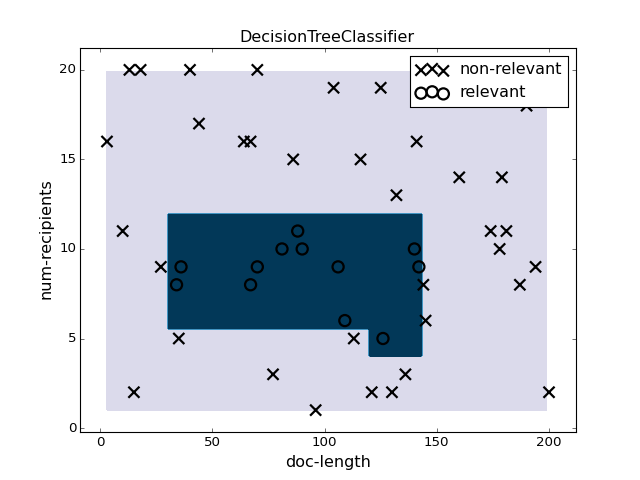(png, hires.png, pdf)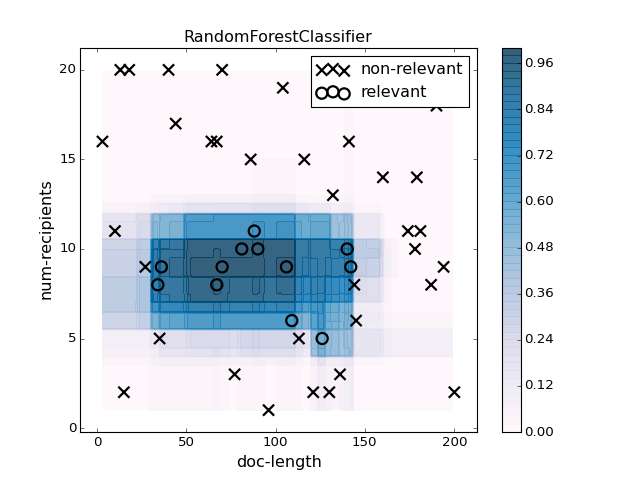(png, hires.png, pdf)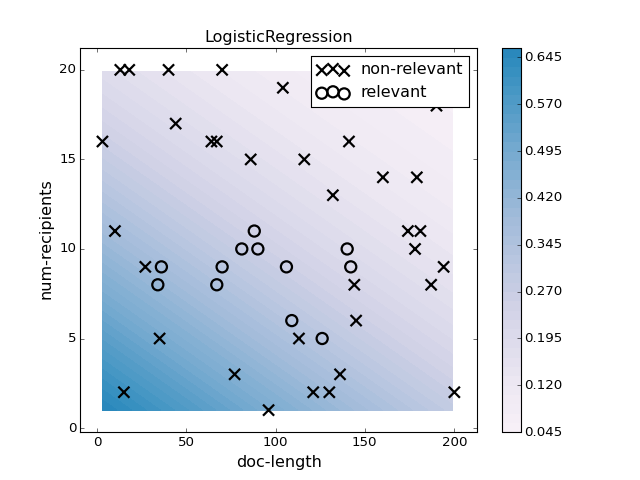(png, hires.png, pdf)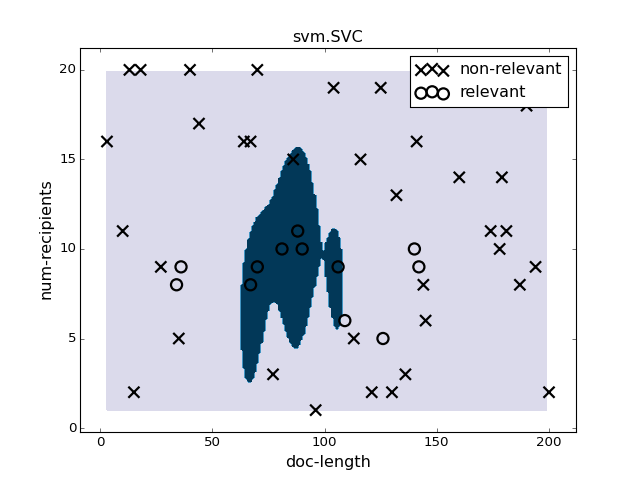(png, hires.png, pdf)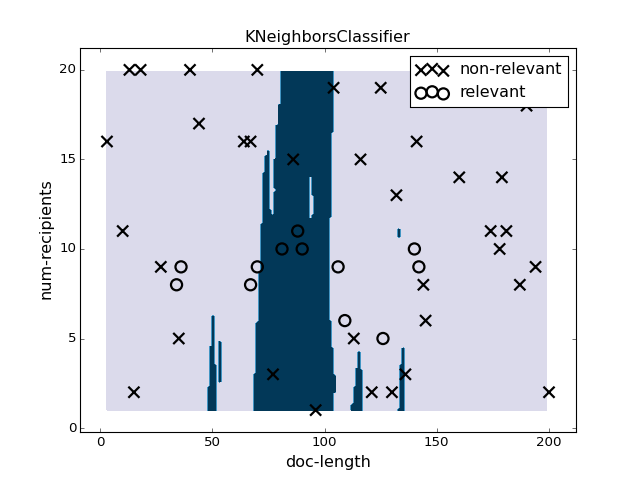(png, hires.png, pdf)

"""
An example script plotting some regressors using prediction_plotter.
"""
import numpy as np
import matplotlib.pylab as pl
pl.ion()

from sklearn.linear_model import LinearRegression
from sklearn.neighbors import KNeighborsRegressor
from sklearn import svm
from sklearn.gaussian_process import GaussianProcess

from rosetta.modeling import prediction_plotter

###############################################################################
# Make training data
###############################################################################
# X data
N = 10 # Number of data points
x1min, x1max, x2min, x2max = 0., 200., 1., 20.
x1 = np.random.randint(x1min, x1max+1, size=N)
x2 = np.random.randint(x2min, x2max+1, size=N)
X = np.c_[x1, x2]

# y data
# y is bigger near the center of the support of X
# noise is added to y
x1mid = (x1max - x1min) / 2.
x2mid = (x2max - x2min) / 2.
center = np.array([x1mid, x2mid])
width1, width2 = x1mid/2, x2mid/2

noise_level = 0.2
product = ((X[:, 0] - center) / width1)**4 + ((X[:, 1] - center) / width2)**4
y = np.exp(- product / 2. ) + noise_level * np.random.randn(N)

###############################################################################
# Initialize the plotter
###############################################################################
plotter = prediction_plotter.RegressorPlotter2D(
x_names=['age', 'height'], y_name='measured-data')

###############################################################################
# Linear Regression
###############################################################################
pl.figure(1)
pl.clf()

clf = LinearRegression().fit(X, y)

plotter.plot(clf, X, y)
pl.title("LinearRegression")
pl.colorbar()

###############################################################################
# K Nearest Neighbors
###############################################################################
pl.figure(2)
pl.clf()

n_neighbors = max(2, N/10)
clf = KNeighborsRegressor(n_neighbors=n_neighbors).fit(X, y)

plotter.plot(clf, X, y)
pl.title("KNeighborsRegressor")
pl.colorbar()

###############################################################################
# Support Vector Regressor (SVR, a.k.a. RVM)
###############################################################################
pl.figure(4)
pl.clf()

clf = svm.SVR(gamma=0.01).fit(X, y)

plotter.plot(clf, X, y)
pl.title("svm.SVR")
pl.colorbar()

###############################################################################
# Gaussian Process Models
###############################################################################
pl.figure(5)
pl.clf()

clf = GaussianProcess(regr='linear', theta0=2).fit(X, y)

plotter.plot(clf, X, y)
pl.title("GaussianProcess")
pl.colorbar()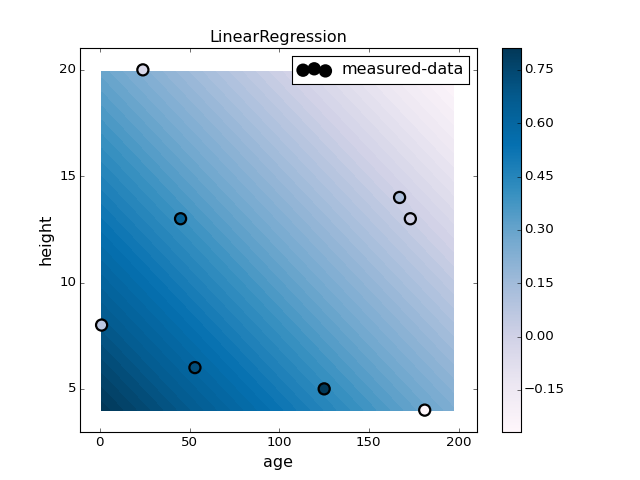(png, hires.png, pdf)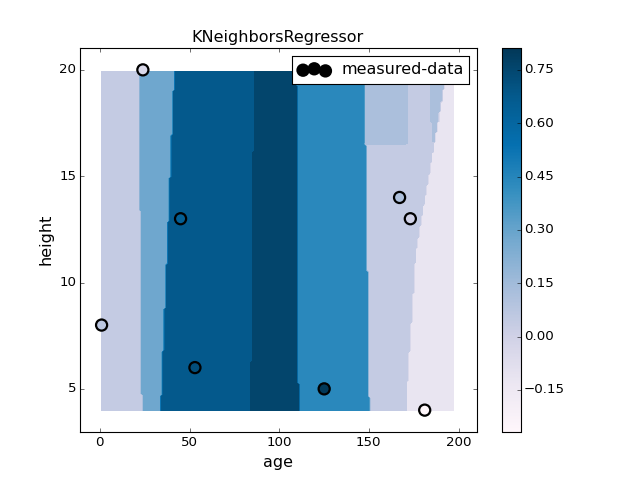(png, hires.png, pdf)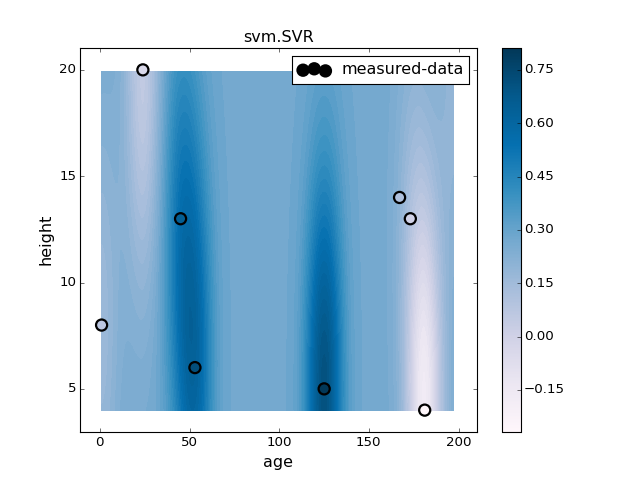(png, hires.png, pdf)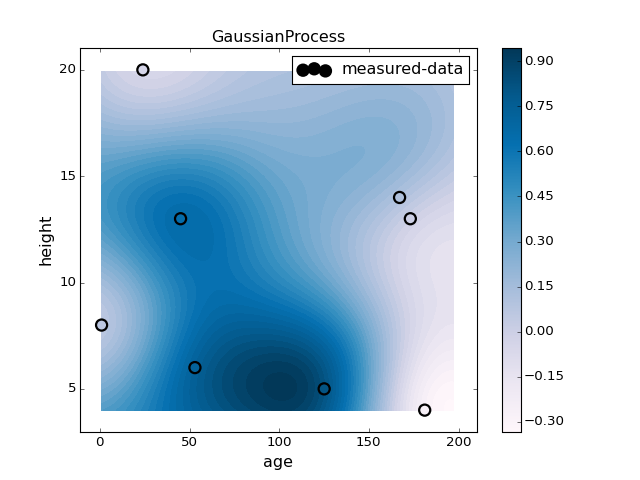(png, hires.png, pdf)

#### eda examples¶

"""
Examples using rosetta.modeling.eda
"""
import pandas as pd
import numpy as np
import matplotlib.pylab as pl

from numpy.random import randn, rand

from rosetta.modeling import eda

###############################################################################
# X-Y plotting
###############################################################################
N = 1000

# Make a linear income vs. age relationship.
age = pd.Series(100 * rand(N))
age.name = 'age'
income = 10 * age + 10 * randn(N)
income.name = 'income'

# The relationship E[Y | X=x] is linear
pl.figure(1); pl.clf()
eda.plot_reducedY_vs_binnedX(age, income)

# Make a sigmoidal P[is_manager | X=x] relationship
def sigmoid(x):
x_st = 5 * (x - x.mean()) / x.std()
return np.exp(x_st) / (1 + np.exp(x_st))

is_manager = (rand(N) < sigmoid(age)).astype('int')
is_manager.name = 'is_manager'
pl.figure(2); pl.clf()
eda.plot_reducedY_vs_binnedX(age, is_manager)

###############################################################################
# Correlation matrix plotting
###############################################################################

# P[has_porsche] is higher for young rich people
has_porsche = (rand(N) < sigmoid(income - 0.5 * age)).astype('int')
has_porsche.name = 'has_porsche'

has_hotwheels = (rand(N) < sigmoid(-age)).astype('int')
has_hotwheels.name = 'has_hotwheels'

all_vars = pd.concat(
[age, income, is_manager, has_porsche, has_hotwheels], axis=1)
corr = all_vars.corr()

fig = pl.figure(3); pl.clf()
eda.plot_corr_grid(corr)

fig = pl.figure(4); pl.clf()
eda.plot_corr_dendrogram(corr)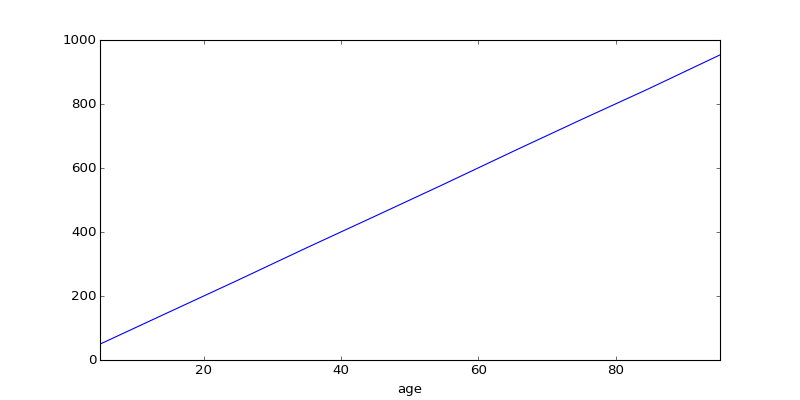(png, hires.png, pdf)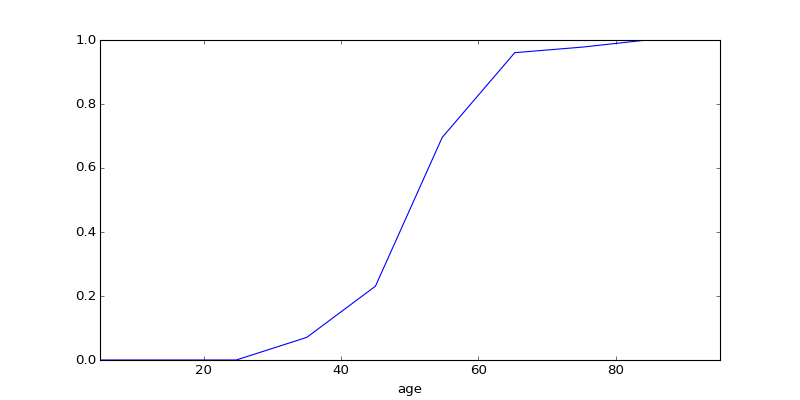(png, hires.png, pdf)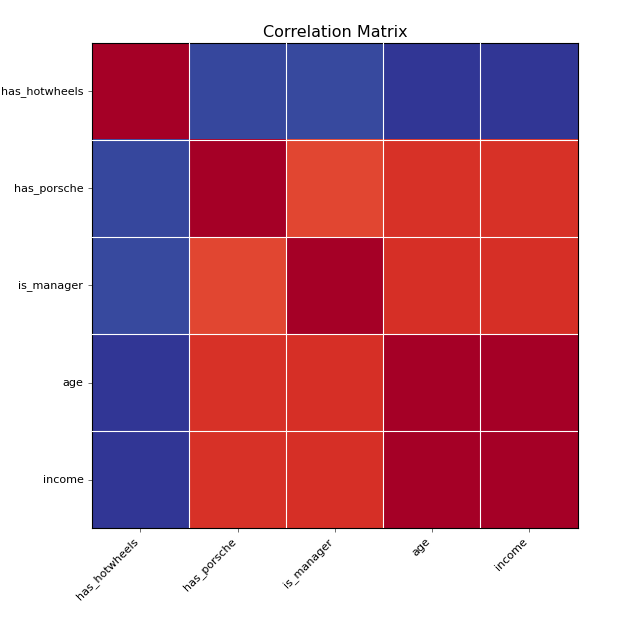(png, hires.png, pdf)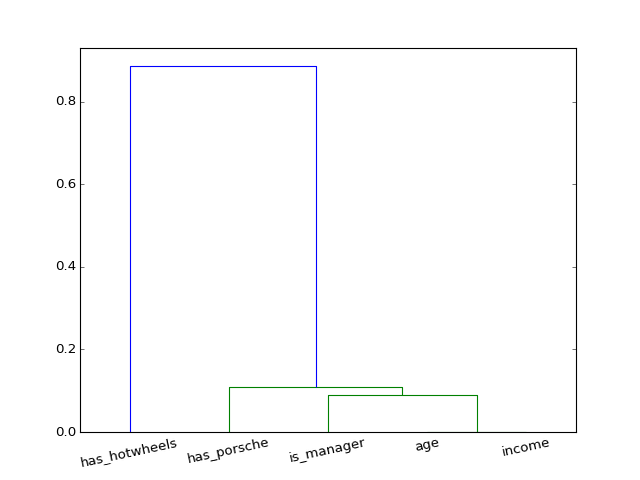(png, hires.png, pdf)